# Multilabel Graph Classification Using Graph Attention Networks

This example shows how to classify graphs that have multiple independent labels using graph attention networks (GATs).

If the observations in your data have a graph structure with multiple independent labels, you can use a GAT  to predict labels for observations with unknown labels. Using the graph structure and available information on graph nodes, GAT uses a masked multihead self-attention mechanism to aggregate features across neighboring nodes, and computes output features or embeddings for each node in the graph. The output features are used to classify the graph usually after employing a readout, or a graph pooling, operation to aggregate or summarize the output features of the nodes.

This example shows how to train a GAT using the QM7-X data set , a collection of graphs that represent 6950 molecules. Each molecule is composed of up to 23 atoms, which are represented as nodes. The data set contains 5 unique atoms: carbon (C), hydrogen (H), nitrogen (N), oxygen (O), and sulfur (S). Three physicochemical properties of the atoms are used as node information: scalar Hirshfeld dipole moments, atomic polarizabilities, and Van der Waals radii. Graph labels are functional groups or specific groups of atoms that play important roles in the formation of molecules. Each functional group represents a subgraph, so a graph can have more than one label or no label if the molecule representing the graph does not have a functional group. This example considers the functional groups CH, CH2, CH3, N, NH, NH2, NOH, and OH.

This figure illustrates the multilabel graph classification workflow in this example.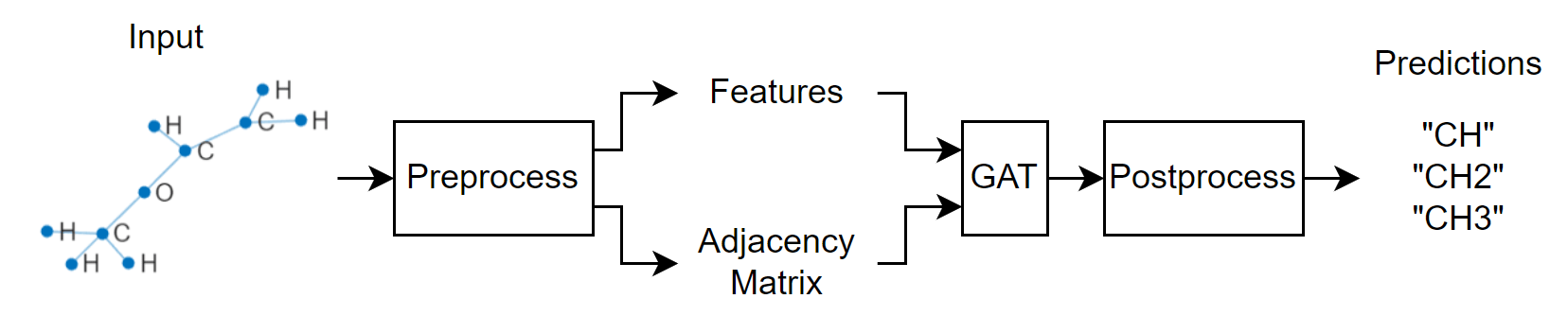Note: Training a GAT is a computationally intensive task. To make the example run quicker, this example skips the training step and loads a pretrained network. To instead train the network, set the doTraining variable to true.

doTraining = false;

Download and unzip the folder containing a processed version of the QM7-X data set from the MathWorks website. The processed version of the data set is a 4.85 MB MAT file consisting of data extracted from the original version downloaded from the QM7-X website.

zipFile = matlab.internal.examples.downloadSupportFile("nnet","data/QM7X.zip"); dataFolder = fileparts(zipFile); unzip(zipFile,dataFolder);

load(fullfile(dataFolder,"QM7X","QM7X.mat"))

View the data.

dataQM7X
dataQM7X = struct with fields: atNUM: [23×6950 double] atXYZ: [3×23×6950 double] hDIP: [23×6950 double] atPOL: [23×6950 double] vdwR: [23×6950 double] 

The data is a structure with 5 fields. The field atNUM contains atomic numbers, atXYZ contains node coordinates, and hDIP, atPOL, and vdwR contain node features. The data consists of a total of 6950 graphs with up to 23 nodes per graph. For graphs that have fewer than 23 nodes, the data is padded with zeros.

### Prepare Data for Training

Extract and concatenate the node features.

features = cat(3,dataQM7X.hDIP,dataQM7X.atPOL,dataQM7X.vdwR); features = permute(features,[1 3 2]);

View the size of the feature data.

size(features)
ans = 1×3 23 3 6950 

Extract the atomic numbers and the coordinates data and use them to build the adjacency matrix data by using the coordinates2Adjacency function, attached to this example as a supporting file. To access this file, open the example as a live script.

atomicNumber = dataQM7X.atNUM; coordinates = dataQM7X.atXYZ; adjacency = coordinates2Adjacency(coordinates,atomicNumber);

View the size of the adjacency data.

size(adjacency)
ans = 1×3 23 23 6950 

Extract the labels using the uniqueFunctionalGroups function, attached to this example as a supporting file. To access this file, open the example as a live script.

labels = uniqueFunctionalGroups(adjacency,atomicNumber);

View the size of the label data.

size(labels)
ans = 1×2 6950 1 

Partition the data into training, validation, and test partitions containing 80%, 10%, and 10% of the data, respectively. To get a random split, use the trainingPartitions function, attached to this example as a supporting file. To access this file, open the example as a live script.

numGraphs = size(adjacency,3); [idxTrain,idxValidation,idxTest] = trainingPartitions(numGraphs,[0.8 0.1 0.1]); featuresTrain = features(:,:,idxTrain); featuresValidation = features(:,:,idxValidation); featuresTest = features(:,:,idxTest); adjacencyTrain = adjacency(:,:,idxTrain); adjacencyValidation = adjacency(:,:,idxValidation); adjacencyTest = adjacency(:,:,idxTest); labelsTrain = labels(idxTrain); labelsValidation = labels(idxValidation); labelsTest = labels(idxTest);

Normalize the features using the mean and variance of the nonzero elements of the training features.

numFeatures = size(featuresTrain,2); muX = zeros(1,numFeatures); sigsqX = zeros(1,numFeatures); for i = 1:numFeatures X = nonzeros(featuresTrain(:,i,:)); muX(i) = mean(X); sigsqX(i) = var(X, 1); end

Normalize the training features, excluding the zero elements that pad the data.

numGraphsTrain = size(featuresTrain,3); for j = 1:numGraphsTrain validIdx = 1:nnz(featuresTrain(:,1,j)); featuresTrain(validIdx,:,j) = (featuresTrain(validIdx,:,j) - muX)./sqrt(sigsqX); end

Normalize the validation features using the same statistics and also exclude the zero elements that pad the data.

numGraphsValidation = size(featuresValidation,3); for j = 1:numGraphsValidation validIdx = 1:nnz(featuresValidation(:,1,j)); featuresValidation(validIdx,:,j) = (featuresValidation(validIdx,:,j) - muX)./sqrt(sigsqX); end

Get the class names from the label data.

classNames = unique(cat(1,labels{:}))
classNames = 8×1 string "CH" "CH2" "CH3" "N" "NH" "NH2" "NOH" "OH" 

Encode the training labels into a binary array of size numObservations-by-numClasses, where numObservations is the number of observations and numClasses is the number of classes. In each row, the nonzero entries correspond to the labels of each observation.

TTrain = zeros(numGraphsTrain,numel(classNames)); for j = 1:numGraphsTrain if ~isempty(labelsTrain{j}) [~,idx] = ismember(labelsTrain{j},classNames); TTrain(j,idx) = 1; end end

View the size of the training target data.

size(TTrain)
ans = 1×2 5560 8 

Visualize the number of graphs per class using a bar graph.

classCounts = sum(TTrain,1); figure bar(classCounts) ylabel("Count") xticklabels(classNames)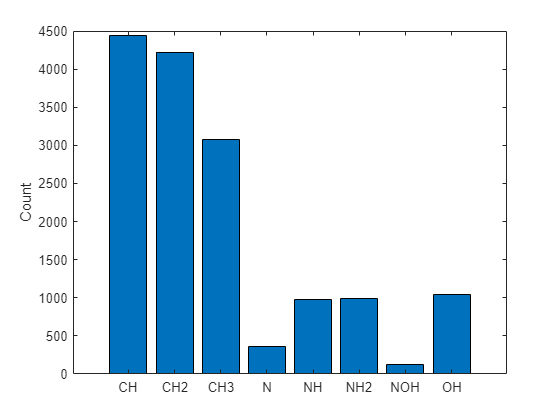Visualize the number of labels per graph using a histogram.

labelCounts = sum(TTrain,2); figure histogram(labelCounts) xlabel("Number of Labels") ylabel("Frequency")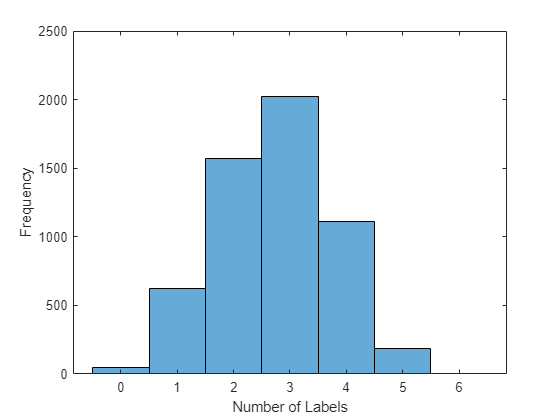The plot shows that a very small percentage of the graphs have zero labels.

Encode the validation labels into a binary array.

TValidation = zeros(numGraphsValidation,numel(classNames)); for j = 1:numGraphsValidation if ~isempty(labelsValidation{j}) [~,idx] = ismember(labelsValidation{j},classNames); TValidation(j,idx) = 1; end end

To train using mini-batches of data, create array datastores for the feature, adjacency, and target training data and combine them.

featuresTrain = arrayDatastore(featuresTrain,IterationDimension=3); adjacencyTrain = arrayDatastore(adjacencyTrain,IterationDimension=3); targetTrain = arrayDatastore(TTrain); dsTrain = combine(featuresTrain,adjacencyTrain,targetTrain);

To make predictions using mini-batches of data, create an array datastore for the validation features and adjacency data and combine them.

featuresValidation = arrayDatastore(featuresValidation,IterationDimension=3); adjacencyValidation = arrayDatastore(adjacencyValidation,IterationDimension=3); dsValidation = combine(featuresValidation,adjacencyValidation);

### Define Model

Define the model. The model takes as input a feature matrix X and an adjacency matrix A and outputs categorical predictions.• The model uses a masked multihead self attention mechanism to aggregate features across the neighborhood of a node, that is, the set of nodes that are directly connected to the node. The mask, which is obtained from the adjacency matrix, is used to prevent attention between nodes that are not in the same neighborhood.

• The model uses ELU nonlinearity, after the first two attention operators, as the activation function.

• To aid convergence, the model uses a skip connection between the last two attention operators.

• To make graph-level predictions using output node features, the model uses averaging to summarize the node features.

• Finally, the model computes independent class probabilities using a sigmoid operation.

#### Initialize Model Parameters

Define the parameters for the each of the operations and include them in a structure.

Create a structure to contain the number of heads for the attention operations.

numHeads = struct;

Specify 3 heads for the first and second attention operations. Specify 5 heads for the third attention operation.

numHeads.attn1 = 3; numHeads.attn2 = 3; numHeads.attn3 = 5;

Create a structure to contain the learnable parameters for the model.

parameters = struct;

Use the format parameters.operationName.parameterName, where parameters is the structure, operationName is the name of the operation (for example, "attn1"), and parameterName is the name of the parameter (for example, "Weights").

Initialize the learnable weights using the initializeGlorot function, attached to this example as a supporting file. To access the function, open the example as a live script.

The attention operation uses two types of weights. Linear weights are used to transform the input features across different attention heads linearly. Attention weights are used to compute attention coefficients at each head. Specify the two weights in a structure with the fields linearWeights and attentionWeights.

Initialize the weights of the first attention operation to have an output size of 96. The input size is the number of channels of the input feature data.

numInputFeatures = size(features,2); numHiddenFeatureMaps = 96; numClasses = numel(classNames); sz = [numInputFeatures numHiddenFeatureMaps]; numOut = numHiddenFeatureMaps; numIn = numInputFeatures; parameters.attn1.weights.linearWeights = initializeGlorot(sz,numOut,numIn); parameters.attn1.weights.attentionWeights = initializeGlorot([numOut 2],1,2*numOut);

Initialize the weights of the second attention operation to have the same output size as the previous multiply operation. The input size is the output size of the previous attention operation.

sz = [numHiddenFeatureMaps numHiddenFeatureMaps]; numOut = numHiddenFeatureMaps; numIn = numHiddenFeatureMaps; parameters.attn2.weights.linearWeights = initializeGlorot(sz,numOut,numIn); parameters.attn2.weights.attentionWeights = initializeGlorot([numOut 2],1,2*numOut);

Initialize the weights of the third attention operation to have an output size matching the number of classes. The input size is the output size of the previous attention operation.

numOutputFeatureMaps = numHeads.attn3*numClasses; sz = [numHiddenFeatureMaps numOutputFeatureMaps]; numOut = numClasses; numIn = numHiddenFeatureMaps; parameters.attn3.weights.linearWeights = initializeGlorot(sz,numOut,numIn); parameters.attn3.weights.attentionWeights = initializeGlorot([numOutputFeatureMaps 2],1,2*numOut);

View the parameters structure.

parameters
parameters = struct with fields: attn1: [1×1 struct] attn2: [1×1 struct] attn3: [1×1 struct] 

View the parameters of the first attention operation.

parameters.attn1.weights
ans = struct with fields: linearWeights: [3×96 dlarray] attentionWeights: [96×2 dlarray] 

#### Define Model Function

Create the function model, defined in the Model Function section of the example, which takes as input the model parameters, the input features and adjacency matrix, and the number of nodes per graph, and returns predictions for the labels.

#### Define Model Loss Function

Create the function modelLoss, defined in the Model Loss Function section of the example, whichhe model parameters, a mini-batch of input features and corresponding adjacency matrix, the number of nodes per graph, and the corresponding encoded targets of the labels, and returns the loss, the gradients of the loss with respect to the learnable parameters, and the model predictions.

### Specify Training Options

Train for 70 epochs with a mini-batch size of 300. Large mini-batches of training data for GATs can cause out-of-memory errors. If your hardware does not have enough memory, then reduce the mini-batch size.

numEpochs = 70; miniBatchSize = 300;

Train using a learning rate of 0.01.

learnRate = 0.01;

To convert prediction probabilities to binary encoded labels, specify a label threshold of 0.5. When the network makes predictions, the network outputs 1 where the probability is greater than or equal to the threshold.

labelThreshold = 0.5;

Validate the model every 210 iterations.

validationFrequency = 210;

### Train Model

Train the model using a custom training loop.

Use minibatchqueue to process and manage mini-batches of training data. For each iteration and mini-batch:

• Use the custom mini-batch preprocessing function preprocessMiniBatch, defined in the Preprocess Mini-Batch Function section of the example to remove zero paddings from the data, compute the number of nodes per graph, and merge multiple graph instances into a single graph instance. The preprocessMiniBatch function returns 4 variables as output. Therefore, set the number of outputs of the minibatchqueue object to 4. By default, the minibatchqueue object sets the number of outputs to the number of variables of the input datastore, which is 3 in this case.

• Cast the data types of the output data to double. The adjacency data is returned as a sparse matrix, and sparse does not support single. By default, the minibatchqueue object casts the data type to single.

• Convert only the feature data to a dlarray object. By default, the minibatchqueue object converts all output data to dlarray objects.

• Train on a GPU if one is available by specifying the output environment of the first output as "auto" and the remaining outputs as "cpu". By default, the minibatchqueue object converts each output to a gpuArray if a GPU is available. Using a GPU requires Parallel Computing Toolbox™ and a supported GPU device. For information on supported devices, see GPU Support by Release (Parallel Computing Toolbox).

mbq = minibatchqueue(dsTrain,4, ... MiniBatchSize=miniBatchSize, ... PartialMiniBatch="discard", ... MiniBatchFcn=@preprocessMiniBatch, ... OutputCast="double", ... OutputAsDlarray=[1 0 0 0], ... OutputEnvironment = ["auto" "cpu" "cpu" "cpu"]);

The modelPredictions function, defined in the Model Predictions section of the example, can be used to obtain model predictions for validating the network. The function makes predictions by iterating over mini-batches of data using the read size property of the datastore object. Set the read size properties of the array datastore holding the validation data to miniBatchSize. You can use any value within your hardware memory allowance.

dsValidation.UnderlyingDatastores{1}.ReadSize = miniBatchSize; dsValidation.UnderlyingDatastores{2}.ReadSize = miniBatchSize;

Initialize the parameters for Adam optimizer.

trailingAvg = []; trailingAvgSq = [];

Train the model.

For each epoch, shuffle the data and loop over the mini-batches.

For each mini-batch:

• Evaluate the model loss and gradients using dlfeval and the modelLoss function.

• Update the network parameters using the adamupdate function.

• Update the training plot.

• If necessary, validate the network using the modelPredictions function. The modelPredictions function takes as input the datastore object dsValidation and the model parameters and returns the model predictions. Calculate the validation loss and update the training plot.

Note: Training a GAT is a computationally intensive task. To make the example run quicker, this example skips the training step and loads a pretrained network. To instead train the network, set the doTraining variable to true.

if doTraining % Initialize the training progress plot. figure C = colororder; lineLossTrain = animatedline(Color=C(2,:)); lineLossValidation = animatedline( ... LineStyle="--", ... Marker="o", ... MarkerFaceColor="black"); ylim([0 inf]) xlabel("Iteration") ylabel("Loss") grid on iteration = 0; start = tic; % Loop over epochs. for epoch = 1:numEpochs % Shuffle data. shuffle(mbq); while hasdata(mbq) iteration = iteration + 1; % Read mini-batches of data. [XTrain,ATrain,numNodes,TTrain] = next(mbq); % Evaluate the model loss and gradients using dlfeval and the % modelLoss function. [loss,gradients,Y] = dlfeval(@modelLoss,parameters,XTrain,ATrain,numNodes,TTrain,numHeads); % Update the network parameters using the Adam optimizer. [parameters,trailingAvg,trailingAvgSq] = adamupdate(parameters,gradients, ... trailingAvg,trailingAvgSq,iteration,learnRate); % Display the training progress. D = duration(0,0,toc(start),Format="hh:mm:ss"); title("Epoch: " + epoch + ", Elapsed: " + string(D)) loss = double(loss); addpoints(lineLossTrain,iteration,loss) drawnow % Display validation metrics. if iteration == 1 || mod(iteration,validationFrequency) == 0 YValidation = modelPredictions(parameters,dsValidation,numHeads); lossValidation = crossentropy(YValidation,TValidation,TargetCategories="independent",DataFormat="BC"); lossValidation = double(lossValidation); addpoints(lineLossValidation,iteration,lossValidation) drawnow end end end else % Load the pretrained parameters. load("parametersQM7X_GAT.mat") end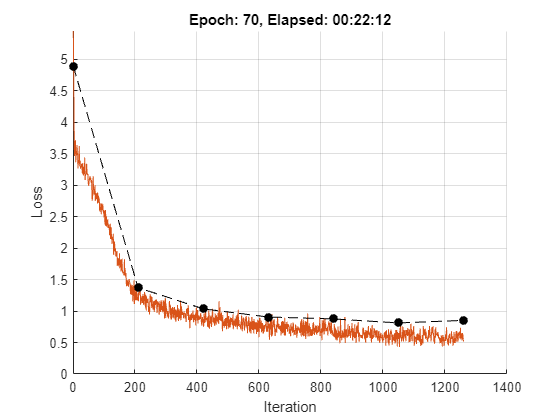### Test Model

Normalize the test features using the statistics of the training features.

numGraphsTest = size(featuresTest,3); for j = 1:numGraphsTest validIdx = 1:nnz(featuresTest(:,1,j)); featuresTest(validIdx,:,j) = (featuresTest(validIdx,:,j) - muX)./sqrt(sigsqX); end

Create array datastores for the test features and adjacency data, setting their ReadSize properties to miniBatchSize, and combine them.

featuresTest = arrayDatastore(featuresTest,IterationDimension=3,ReadSize=miniBatchSize); adjacencyTest = arrayDatastore(adjacencyTest,IterationDimension=3,ReadSize=miniBatchSize); dsTest = combine(featuresTest,adjacencyTest);

Encode the test labels into a binary array.

TTest = zeros(numGraphsTest,numel(classNames)); for j = 1:numGraphsTest if ~isempty(labelsTest{j}) [~,idx] = ismember(labelsTest{j},classNames); TTest(j,idx) = 1; end end

Use the modelPredictions function to make predictions on the test data.

predictions = modelPredictions(parameters,dsTest,numHeads);

Convert prediction probabilities to binary encoded labels using a label threshold of 0.5, which is the same as the label threshold labelThreshold, used when training and validating the model.

predictions = double(gather(extractdata(predictions))); YTest = double(predictions >= 0.5);

Evaluate the performance by calculating the F-score using the fScore function, defined in the F-Score Function section of the example.

The fScore function uses a weighting parameter beta to place greater value on either precision or recall. Precision is the ratio of true positive results to all positive results, including those that are incorrectly predicted as positive, and recall is the ratio of true positive results to all actual positive samples.

Calculate the F-score using three weighting parameters:

• 0.5 — Precision is twice as important as recall.

• 1 — Precision and recall are equally important. Use this value to monitor the performance of the model during training and validation.

• 2 — Recall is twice as important as precision.

scoreWeight = [0.5 1 2]; for i = 1:3 scoreTest(i) = fScore(YTest,TTest,scoreWeight(i)); end

View the scores in a table.

scoreTestTbl = table; scoreTestTbl.Beta = scoreWeight'; scoreTestTbl.FScore = scoresTest'
scoreTestTbl=3×2 table Beta FScore ____ ______ 0.5 0.9492 1 0.9475 2 0.9458 

Visualize the confusion chart for each class.

figure tiledlayout("flow") for i = 1:numClasses nexttile confusionchart(YTest(:,i),TTest(:,i)); title(classNames(i)) end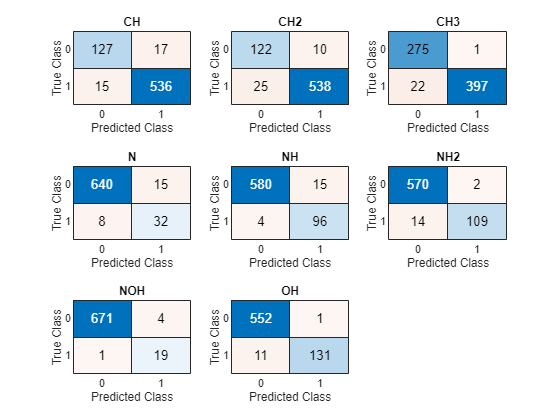Visualize the receiver operating characteristics (ROC) curves for each class.

The ROC curve plots the true positive rates versus false positive rates and illustrates the performance of the model at all labeling thresholds. The true positive rate is the ratio of true positive results to all actual positive samples, including those that the model incorrectly predicts as negative. The false positive rate is the ratio of false positive results to all actual negative samples, including those that are incorrectly predicted as positive. The area under the curve (AUC) provides an aggregate measure of performance across all possible labeling thresholds.

For each class:

• Compute the true positive rates and false positive rates using the roc function.

• Calculate the AUC using the trapz function.

• Plot the ROC curve and display the AUC. Also plot the ROC curve of a random, or no-skill, model that makes random predictions, or always predicts the same result.

figure tiledlayout("flow") for i = 1:numClasses currentTargets = TTest(:,i)'; currentPredicitons = predictions(:,i)'; [truePositiveRates,falsePositiveRates] = roc(currentTargets,currentPredicitons); AUC = trapz(falsePositiveRates,truePositiveRates); nexttile plot(falsePositiveRates,truePositiveRates, ... falsePositiveRates,falsePositiveRates,"--",LineWidth=0.7) text(0.075,0.75,"\bf AUC = "+num2str(AUC),FontSize=6.75) xlabel("FPR") ylabel("TPR") title(classNames(i)) end lgd = legend("ROC Curve - GAT", "ROC Curve - Random"); lgd.Layout.Tile = numClasses+1;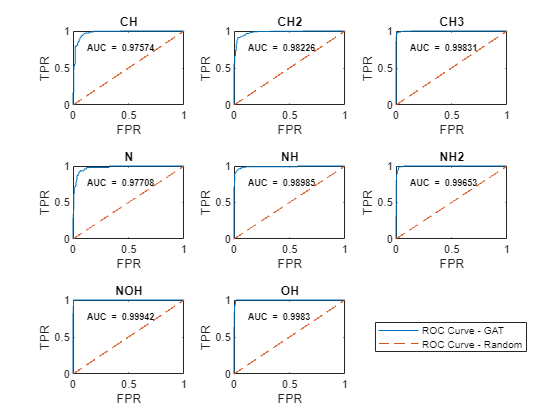### Predict Using New Data

Load the preprocessed QM7X sample data.

load(fullfile(dataFolder,"QM7X","preprocessedQM7XSample.mat"))

Get the adjacency matrix and node features from the sample data.

adjacencyMatrixSample = dataSample.AdjacencyMatrix; featuresSample = dataSample.Features;

View the number of nodes in the graph.

numNodesSample = size(adjacencyMatrixSample,1)
numNodesSample = 10 

Extract the graph data. To compute the attention scores, remove added self-connections from the adjacency matrix, then use the matrix to construct the graph.

A = adjacencyMatrixSample - eye(numNodesSample); G = graph(A);

Map the atomic numbers to symbols using the atomicSymbol function, attached to this example as a supporting file. To access this function, open the example as a live script.

atomicNumbersSample = dataSample.AtomicNumbers; [symbols,symbolsCount] = atomicSymbol(atomicNumbersSample);

Display the graph in a plot, using the mapped symbols as node labels.

figure plot(G,NodeLabel=symbols,LineWidth= 0.75,Layout="force") title("Sample Molecule")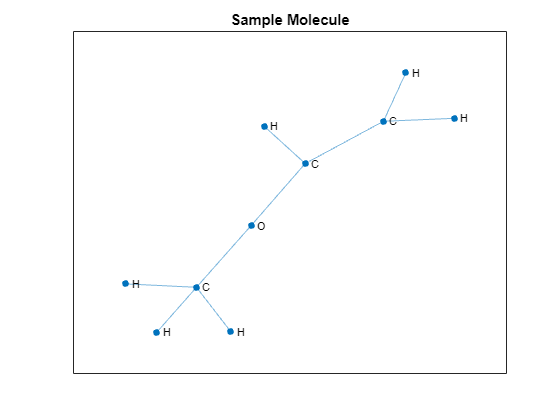Convert the features to a dlarray object.

XSample = dlarray(featuresSample);

If a GPU is available, convert the input data to gpuArray.

if canUseGPU XSample = gpuArray(XSample); end

Make predictions using the model function. Also obtain the attention scores computed by the attention operator in the model.

[YSample,attentionScores] = model(parameters,XSample,adjacencyMatrixSample,numNodesSample,numHeads);

Convert prediction probabilities to binary encoded labels.

YSample = gather(extractdata(YSample)); YSample = YSample >= 0.5;

Convert the predicted binary labels to actual labels.

predictionsSample = classNames(YSample)
predictionsSample = 3×1 string "CH" "CH2" "CH3" 

Visualize the attention scores.

Create a heat map of the attention scores per head in the final attention operation of the model using heatmap.

attention3Scores = double(gather(extractdata(attentionScores.attn3))); numHeadsAttention3 = numHeads.attn3; figure tiledlayout("flow") for i = 1:numHeadsAttention3 nexttile heatmap(symbolsCount,symbolsCount,attention3Scores(:,:,i),ColorScaling="scaledrows",Title="Head "+num2str(i)) end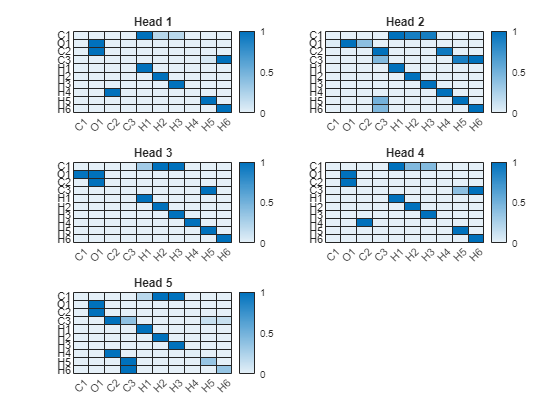The x and y values in the attention maps correspond to the node labels in the plot below.

figure plot(G,NodeLabel=symbolsCount,LineWidth= 0.75,Layout="force")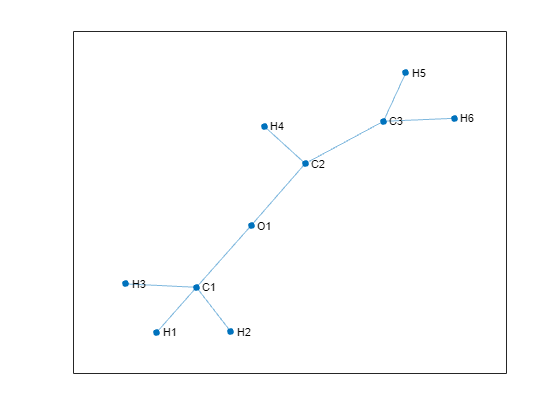The color map intensity signifies the relative level of attention that the node at a particular row index gives to the node at a particular column index. For example, at head 1, the first carbon atom C1 gives an almost equal level of attention to the first three hydrogen atoms H1, H2, and H3, and it gives little or no attention to itself, the oxygen atom O1, which it is also connected to, or to all the other atoms with which it shares no connection.

### Model Function

The model function takes as inputs the model parameters parameters, the feature matrix X, the adjacency matrix A, the number of nodes per graph numNodes, and the number of heads numHeads, and returns the predictions and the attention scores.

The function:

• Computes the graph attention at each layer using the attention function defined in the Attention Function section of the example.

• Uses ELU nonlinearity, using the elu function defined in the ELU Function section of the example as the activation function for the first two layers.

• Employs a residual connection in the hidden layer to aid convergence.

• Uses the globalAveragePool function, defined in the Global Average Pool Function section of the example, to perform the readout operation after the final layer.

• Uses the sigmoid function to compute the label predictions as independent class probabilities.

function [Y,attentionScores] = model(parameters,X,A,numNodes,numHeads) weights = parameters.attn1.weights; numHeadsAttention1 = numHeads.attn1; Z1 = X; [Z2,attentionScores.attn1] = attention(Z1,A,weights,numHeadsAttention1,"cat"); Z2 = elu(Z2); weights = parameters.attn2.weights; numHeadsAttention2 = numHeads.attn2; [Z3,attentionScores.attn2] = attention(Z2,A,weights,numHeadsAttention2,"cat"); Z3 = elu(Z3) + Z2; weights = parameters.attn3.weights; numHeadsAttention3 = numHeads.attn3; [Z4,attentionScores.attn3] = attention(Z3,A,weights,numHeadsAttention3,"mean"); Z4 = globalAveragePool(Z4,numNodes); Y = sigmoid(Z4); end

### Model Loss Function

The modelLoss function takes as inputs the model parameters parameters, the feature matrix X, the adjacency matrix adjacencyTrain, the number of nodes per graph numNodes, the binary encoded labels T, and the number of heads numHeads, and returns the gradients of the loss with respect to the model parameters, the corresponding loss, and the model predictions.

function [loss,gradients,Y] = modelLoss(parameters,X,adjacencyTrain,numNodes,T,numHeads) Y = model(parameters,X,adjacencyTrain,numNodes,numHeads); loss = crossentropy(Y,T,TargetCategories="independent",DataFormat="BC"); gradients = dlgradient(loss,parameters); end

### Preprocess Mini-batch Function

The preprocessMiniBatch function takes as inputs the feature data featureData, the adjacency data adjacencyData, and the target data targetData, and merges mini-batches of different graph instances into a single graph instance.

Note that the target data is an optional input argument to the function.

For each graph, the function:

• Calculates the number of nodes and concatenates the data.

• Removes zero paddings from the adjacencyData input and concatenates the data by building a sparse block-diagonal matrix so that the nodes in different graph instances do not interact, that is, they are not regarded as neighbors. Each block in the matrix corresponds to the adjacency matrix of one graph instance.

• Removes zero paddings from the featureData input and concatenates the data.

function [features,adjacency,numNodes,target] = preprocessMiniBatch(featureData,adjacencyData,targetData) % Extract feature and adjacency data from their cell array and concatenate the % data along the third (batch) dimension featureData = cat(3,featureData{:}); adjacencyData = cat(3,adjacencyData{:}); % Extract target data if it exists if nargin > 2 target = cat(1,targetData{:}); end adjacency = sparse([]); features = []; numNodes = []; for i = 1:size(adjacencyData, 3) % Get the number of nodes in the current graph numNodesInGraph = nnz(featureData(:,1,i)); numNodes = [numNodes; numNodesInGraph]; % Get the indices of the actual nonzero data validIdx = 1:numNodesInGraph; % Remove zero paddings from adjacencyData tmpAdjacency = adjacencyData(validIdx, validIdx, i); % Add self connections tmpAdjacency = tmpAdjacency + eye(size(tmpAdjacency)); % Build the adjacency matrix into a block diagonal matrix adjacency = blkdiag(adjacency, tmpAdjacency); % Remove zero paddings from featureData tmpFeatures = featureData(validIdx, :, i); features = [features; tmpFeatures]; end end

### F-Score Function

The fScore function calculates the micro-average F-score, which measures the model accuracy on the data using the precision and the recall.

The micro-average precision can be defined as

$\text{precision}=\frac{\sum _{n=1}^{N}\sum _{c=1}^{C}{Y}_{nc}×{T}_{nc}}{\sum _{n=1}^{N}\sum _{c=1}^{C}\left({Y}_{nc}×{T}_{nc}\right)+\left({Y}_{nc}×\left(1-{T}_{nc}\right)\right)}$,

where Y is encoded binary predictions, T is encoded binary targets, N is the number of observations, and C is the number of classes.

The micro-average recall can be defined as

$\text{recall}=\frac{\sum _{n=1}^{N}\sum _{c=1}^{C}{Y}_{nc}×{T}_{nc}}{\sum _{n=1}^{N}\sum _{c=1}^{C}{T}_{nc}}$,

and the micro-average F-Score is defined as:

$\left(1+{\beta }^{2}\right)×\frac{\text{precision}×\text{recall}}{\left({\beta }^{2}×\text{precision}\right)+\text{recall}}$,

where $\beta$ is a weighting parameter that is used to place greater value on either the precision or the recall.

function score = fScore(predictions,targets,beta) truePositive = sum(predictions .* targets,"all"); falsePositive = sum(predictions .* (1-targets),"all"); % Precision precision = truePositive/(truePositive + falsePositive); % Recall recall = truePositive/sum(targets,"all"); % FScore if nargin == 2 beta = 1; end score = (1+beta^2)*precision*recall/(beta^2*precision+recall); end

### Model Predictions Function

The modelPredictions function takes as inputs the model parameters parameters, the datastore object ds holding the features and adjacency data, and the number of heads numHeads, and returns the model predictions by iterating over mini-batches of data and preprocessing each mini-batch using the preprocessMiniBatch function.

function Y = modelPredictions(parameters,ds,numHeads) Y = []; reset(ds) while hasdata(ds) data = read(ds); featureData = data(:,1); adjacencyData = data(:,2); [features,adjacency,numNodes] = preprocessMiniBatch(featureData,adjacencyData); X = dlarray(features); minibatchPred = model(parameters,X,adjacency,numNodes,numHeads); Y = [Y;minibatchPred]; end end

### Attention Function

The attention function computes node features using masked multihead self-attention.

Given input node features ${Z}_{l}$ of dimension $N×F$, where $N$ is the number of nodes and $F$ is the number of input features, at layer $l$, the attention function computes the output node features ${Z}_{l+1}$ of dimension $N×K{F}^{\prime }$, where $K{F}^{\prime }$ is the number of output features of the layer, as follows:

1. Using $K$ independent heads, it computes linear transformations ${H}^{k}$, for $k=1,...,K$, of the input features using weight matrices ${W}_{l}^{k}$ of dimensions $F×{F}^{\prime }$. That is, ${H}^{k}={Z}_{l}{W}_{l}^{k}$.

2. It uses an attention mechanism to compute the scalar attention coefficients ${\epsilon }_{nm}^{k}={a}^{k}\left({h}_{n}^{k},{h}_{m}^{k}\right)$ for all node pairs $\left(n,m\right)$, where ${h}_{n}^{k}$ is a vector of dimension $1×{F}^{\prime }$ corresponding to the nth row of matrix ${H}^{k}$. The attention coefficient ${\epsilon }_{nm}^{k}$ represents the importance of node $m$ to node $n$ at head $k$. The attention mechanism $a$ can be a dot product operation of features ${h}_{n}$ and ${h}_{m}$, or a neural network. This example follows the approach in , where features ${h}_{n}$ and ${h}_{m}$ are concatenated and the attention mechanism is a single-layer neural network with weights array ${\theta }_{l}^{k}$, consisting of $2{F}^{\prime }$ elements, that is shared across all nodes, followed by leaky-ReLU nonlinearity.

3. To prevent attention between nodes that are not directly connected, the GAT implements masked attention by using the graph structure, defined by the adjacency matrix, to compute a mask such that the masked attention coefficients ${\underset{}{\overset{‾}{\epsilon }}}_{nm}=-\mathrm{inf}$ for all $m\notin {\mathcal{N}}_{n}$, where ${\mathcal{N}}_{n}$ is the neighborhood of node $n$, including $n$, and ${\underset{}{\overset{‾}{\epsilon }}}_{nm}={\epsilon }_{nm}$ for all $m\in {\mathcal{N}}_{n}$.

4. Attention scores are computed by normalizing the masked attention coeffiecients using a softmax operation. That is, the attention score ${\alpha }_{nm}^{k}=\frac{\mathrm{exp}\left({\underset{}{\overset{‾}{\epsilon }}}_{nm}^{k}\right)}{\sum _{j\in {\mathcal{N}}_{n}}\mathrm{exp}\left({\underset{}{\overset{‾}{\epsilon }}}_{nj}^{k}\right)}$.

5. The attention scores are then used to obtain the output features of the layer as a linear combination of transformations ${H}^{k}$. That is, given a $N×N$matrix of attention scores ${\alpha }^{k}$ computed at head $k$, output features ${Z}_{l+1}^{k}$ from attention head $k$ are obtained as ${Z}_{l+1}^{k}={\alpha }^{k}{H}^{k}$.

6. The output features from each attention head are aggregated, either by concatenation, that is, ${Z}_{l+1}=\left[{Z}_{l+1}^{1},...,{Z}_{l+1}^{K}\right]$, or by averaging, that is ${Z}_{l+1}=\frac{1}{K}\sum _{k=1}^{K}{Z}_{l+1}^{k}$, to give the output features of the layer.

The attention function takes as inputs the feature matrix inputFeatures, adjacency matrix adjacency, learnable parameters weights, the number of heads numHeads, and aggregation method for the multiple attention heads aggregation, and computes and returns features using masked multihead self attention.

The function splits the learnable parameters into multiple heads, and for each attention head, it:

• Computes a linear transformation of the input features.

• Computes attention coefficients using linearly transformed features.

• Normalizes linearly transformed features using normalized masked attention coefficients.

Finally, it aggregates the output features of different attention heads.

function [outputFeatures,normAttentionCoeff] = attention(inputFeatures,adjacency,weights,numHeads,aggregation) % Split weights with respect to the number of heads and reshape the matrix to a 3-D array szFeatureMaps = size(weights.linearWeights); numOutputFeatureMapsPerHead = szFeatureMaps(2)/numHeads; linearWeights = reshape(weights.linearWeights,[szFeatureMaps(1), numOutputFeatureMapsPerHead, numHeads]); attentionWeights = reshape(weights.attentionWeights,[numOutputFeatureMapsPerHead, 2, numHeads]); % Compute linear transformations of input features value = pagemtimes(inputFeatures,linearWeights); % Compute attention coefficients query = pagemtimes(value, attentionWeights(:, 1, :)); key = pagemtimes(value, attentionWeights(:, 2, :)); attentionCoefficients = query + permute(key,[2, 1, 3]); attentionCoefficients = leakyrelu(attentionCoefficients,0.2); % Compute masked attention coefficients mask = -10e9 * (1 - adjacency); attentionCoefficients = attentionCoefficients + mask; % Compute normalized masked attention coefficients normAttentionCoeff = softmax(attentionCoefficients,DataFormat = "BCU"); % Normalize features using normalized masked attention coefficients headOutputFeatures = pagemtimes(normAttentionCoeff,value); % Aggregate features from multiple heads if strcmp(aggregation, "cat") outputFeatures = headOutputFeatures(:,:); else outputFeatures = mean(headOutputFeatures,3); end end

### ELU Function

The elu function implements the ELU activation function defined as

$\mathit{f}\left(\mathit{x}\right)=\left\{\begin{array}{ll}\mathit{x},& \mathit{x}\ge 0\\ \alpha \left(\mathrm{exp}\left(\mathit{x}\right)-1\right),& \mathit{x}<0\end{array}$

The function uses $\alpha =1.$

function y = elu(x) y = max(0, x) + (exp(min(0, x)) -1); end

### Global Average Pool Function

The globalAveragePool function takes as inputs a feature representation inFeatures and the number of nodes per graph numNodes, and returns an output feature representation for each graph by averaging the input features with respect to the number of nodes per graph.

For a graph $G$ with ${N}_{G}$ nodes, the function computes output features for the graph as ${y}_{G}=\frac{1}{{N}_{G}}\sum _{n=1}^{{N}_{G}}{z}_{n}$, where ${z}_{n}$ is a $1×{F}^{\prime }$ vector, corresponding to the nth row of a ${N}_{G}×{F}^{\prime }$ submatrix of input features of the graph, and ${F}^{\prime }$ is the number of input features.

function outFeatures = globalAveragePool(inFeatures,numNodes) numGraphs = numel(numNodes); numFeatures = size(inFeatures, 2); outFeatures = zeros(numGraphs,numFeatures,"like",inFeatures); startIdx = 1; for i = 1:numGraphs endIdx = startIdx + numNodes(i) - 1; idx = startIdx:endIdx; outFeatures(i,:) = mean(inFeatures(idx,:)); startIdx = endIdx + 1; end end

### References

1. Veličković, Petar, Guillem Cucurull, Arantxa Casanova, Adriana Romero, Pietro Liò, and Yoshua Bengio. “Graph Attention Networks." Preprint, submitted February 4, 2018. https://arxiv.org/abs/1710.10903.

2. Hoja, Johannes, Leonardo Medrano Sandonas, Brian Ernst, Alvaro Vazquez-Mayagoitia, Robert A. DiStasio, and Alexandre Tkatchenko. “QM7-X: A Comprehensive Dataset of Quantum-Mechanical Properties Spanning the Chemical Space of Small Organic Molecules.” Zenodo, November 23, 2020. https://doi.org/10.5281/ZENODO.3905360.